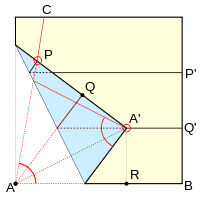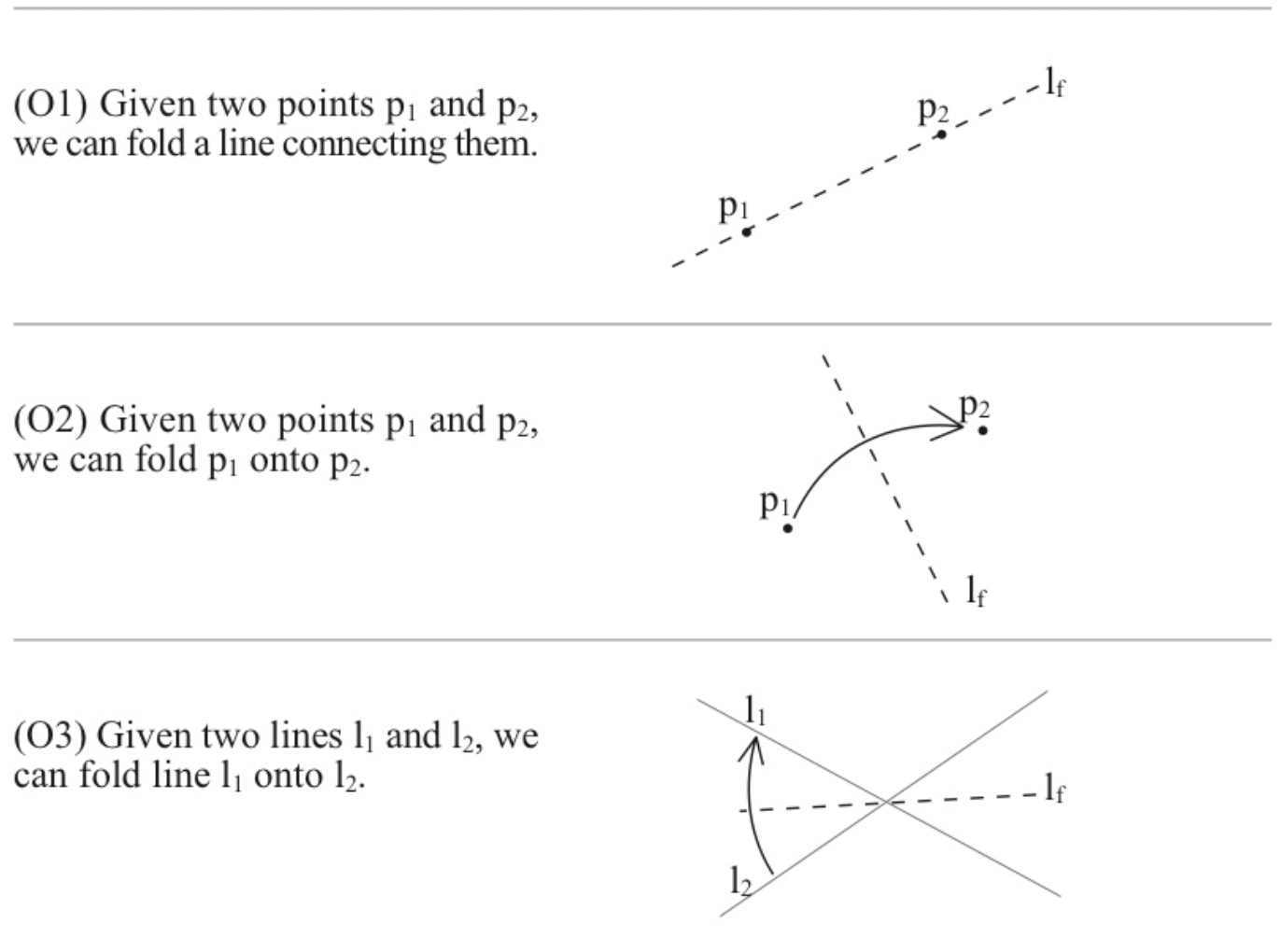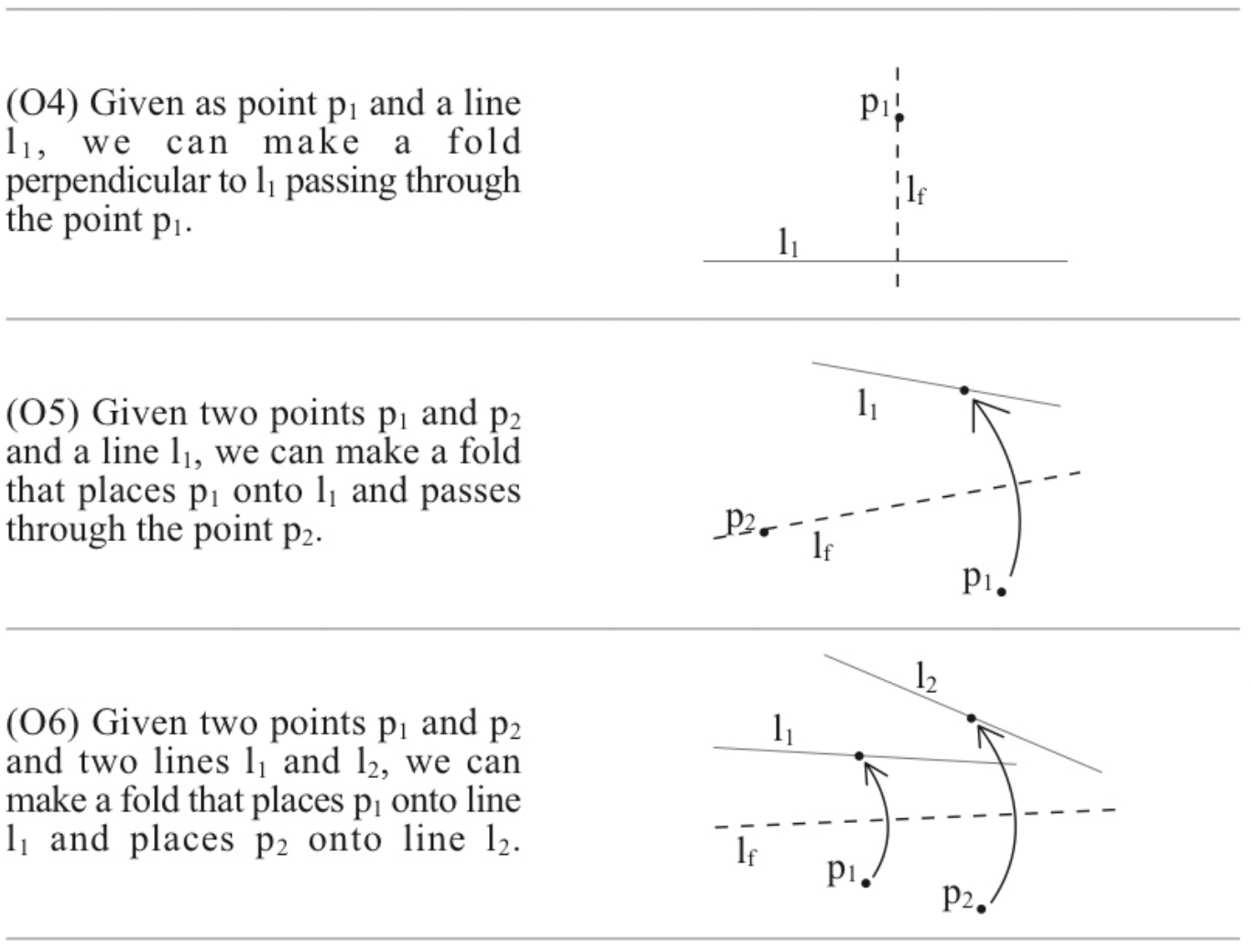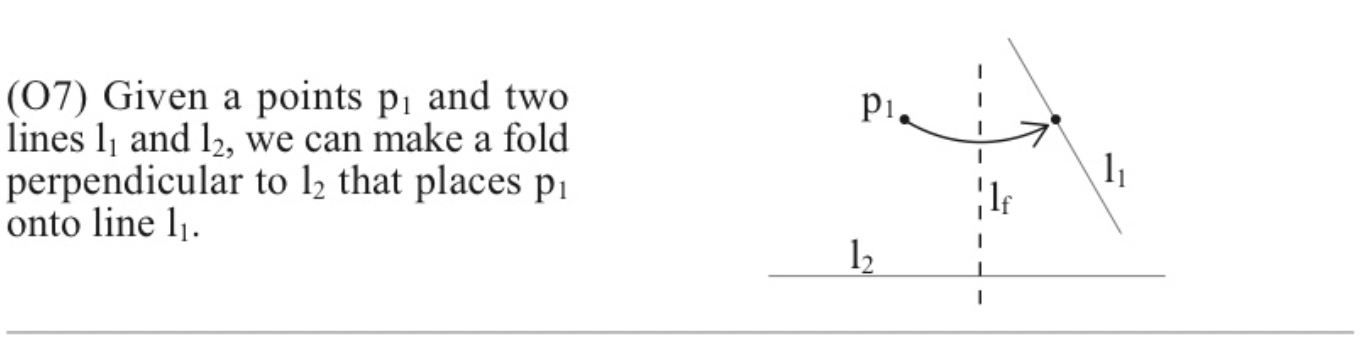#### Origami in Mathematics

by Michael Guenette on Mar 23, 2022All of mathematics is built upon a solid foundation of fundamental mathematical facts. These building blocks allow subsequent ideas to be hypothesized and in many cases proven, adding to the never ending list of theorems and definitions taught in schools today.

For Geometry, these fundamental axioms were formalized by the Greek mathematician Euclid in Alexandria around 300 BC in a series of 13 books known as “Euclid’s Elements”. Early in the book he postulated that the following 5 axioms form the basis of all geometrical constructions, and most Geometry is built from there.

1. "To draw a straight line from any point to any point."
2. "To produce [extend] a finite straight line continuously in a straight line."
3. "To describe a circle with any centre and distance [radius]."
4. "That all right angles are equal to one another."
5. The parallel postulate: "That, if a straight line falling on two straight lines make the interior angles on the same side less than two right angles, the two straight lines, if produced indefinitely, meet on that side on which are the angles less than the two right angles."

These axioms are mostly built on the idea that Geometrical figures are constructed with a straightedge and a compass. Using only those tools there are many constructions and proofs that can be completed, but what if we used a different medium to build this foundation? What if we resorted to the folding of paper to make lines and angles rather than a pencil? It turns out that all previous constructions are possible, and then some. That’s right, Origami (the art of folding paper) can be used to prove mathematical theorems.

We begin this Origametric theory with a set of 7 basic facts called the Huzita–Hatori axioms, named after those who discovered them. These define the list of possible ways that a fold can be created:Using these basic constructions, we can prove anything that is constructible using a straightedge and compass. We also get the ability to create new constructions that were not possible with the conventional methods. Many of these proofs and constructions are quite complicated, but there is one simple construction that is now possible from these axioms: the trisection of an angle.

Using a standard straightedge and compass it is very easy to bisect an angle (split it into two equal angles), but there is no possible way to perform a trisection (splitting it into three equal angles). However, using only the 7 axioms listed above we can fold paper in such a way that any angle can be divided into three equal angles. (I leave this as an exercise for the reader.)

Origami has been around for hundreds (if not thousands) of years. Its origin is not well documented, which is ironic since there is always paper around when origami is involved. The discovery of these axioms has led to some amazing enhancements in the world of origami over the last few decades, and it will only get better from here.